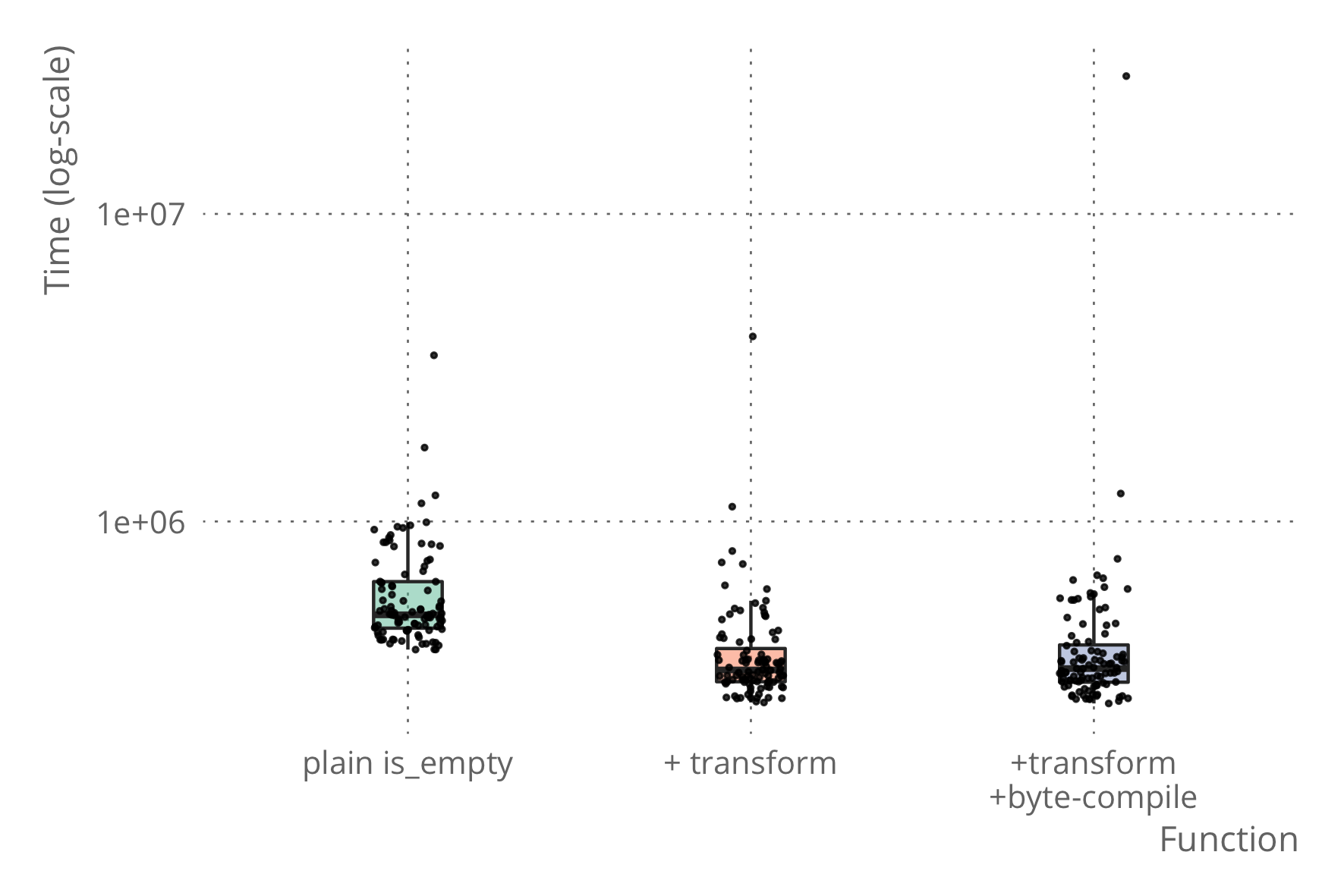# The Working R Programmer

Tips and tricks for serious software development in R

# Transforming functions with cases calls

The issue with byte-compilation I wrote about yesterday can indeed be fixed by transforming functions that call `cases`. And that was very easy to implement since I already had all the bits and pieces I needed for it from the `tailr` transformations.

To remind you, the issue was with byte-compiling functions that use the `pmatch` DSL in a `cases` call. For example, this function that tests if a tree is a leaf:

``````library(pmatch)

tree := L(num) | T(left : tree, right : tree)

is_leaf <- function(tree) {
cases(tree,
L(x) -> TRUE,
otherwise -> FALSE)
}``````

The function works as intended so in usual code you will not run into any issues with it.

``is_leaf(L(1))``
``````##  TRUE
``````
``is_leaf(T(L(1),L(2)))``
``````##  FALSE
``````

If you use it in a package, though, it will (by default) be byte-compiled, and the byte-compiler does not approve of assigning to `TRUE` or `FALSE`.

``compiler::cmpfun(is_leaf)``
``````## Error: bad assignment: 'TRUE <- L(x)'
``````

There is a similar issue if you want to throw errors with `stop`. This works fine

``````get_left <- function(tree) {
cases(
tree,
T(left, right) -> left,
otherwise ->
stop("There is no left tree!", call. = FALSE)
)
}``````
``get_left(T(L(1),L(2)))``
``````## L(num = 1)
``````
``get_left(L(1))``
``````## Error: There is no left tree!
``````

but the byte-compiler will complain

``compiler::cmpfun(get_left)``
``````## Error: bad assignment: 'stop("There is no left tree!", call. = FALSE) <- otherwise'
``````

## Transforming functions

We can get rid of this problem by transforming the functions that call `cases`. I already do this when I combine `cases` with `tailr` to make functions tail-recursive, so I already had this function:

``````transform_cases_call <- function(expr) {
stopifnot(rlang::call_name(expr) == "cases")

args <- rlang::call_args(expr)
value <- args[]
patterns <- args[-1]
eval(rlang::expr(cases_expr(!!value, !!!patterns)))
}``````

It takes a `call` object—the representation of a function-call when you manipulate R expressions—and returns a series of `if`-`else`-expressions, computed by the `cases_expr` function.

So far, I have only used this with `tailr` and its user-transformation plugin

``attr(cases, "tailr_transform") <- transform_cases_call``

but now I use it for this transformation function:

``````transform_cases_function_rec <- function(expr) {
if (rlang::is_atomic(expr) || rlang::is_pairlist(expr) ||
rlang::is_symbol(expr) || rlang::is_primitive(expr)) {
expr
} else {
stopifnot(rlang::is_lang(expr))
call_args <- rlang::call_args(expr)
for (i in seq_along(call_args)) {
expr[[i + 1]] <- transform_cases_function_rec(call_args[[i]])
}
if (rlang::call_name(expr) == "cases") {
expr <- transform_cases_call(expr)
}
expr
}
}

transform_cases_function <- function(fun) {
if (!rlang::is_closure(fun)) {
err <- simpleError("Function must be a closure to be transformed")
stop(err)
}
body(fun) <- transform_cases_function_rec(body(fun))
fun
}``````

The way I test if a call to `cases` is actually a call to `pmatch::cases` is a bit dodgy. It only works if the `cases` that is in scope is the right one. To fix this, I have to carry the environment along in the recursions, but I haven’t implemented this yet.

Anyway, using this function, you can automatically rewrite a function from using calls to `cases` to using `if`-statements.

``````is_leaf_tr <- transform_cases_function(is_leaf)
is_leaf_tr``````
``````## function (tree)
## {
##     if (!rlang::is_null(..match_env <- pmatch::test_pattern(tree,
##         L(x))))
##         with(..match_env, TRUE)
##     else if (!rlang::is_null(..match_env <- pmatch::test_pattern(tree,
##         otherwise)))
##         with(..match_env, FALSE)
## }
``````

## Results

After we have translated a function, there is no more alternative DSL syntax, and that satisfy the byte-compiler.

``is_leaf_tr_bc <- compiler::cmpfun(is_leaf_tr)``

As an added benefit, the transformed function is a bit faster than the one that calls `cases`, and the byte-compiled function is a little faster still.

``````microbenchmark::microbenchmark(
is_leaf(L(1)), is_leaf_tr(L(1)), is_leaf_tr_bc(L(1))
)``````
``````## Unit: microseconds
##                 expr     min       lq     mean  median       uq      max
##        is_leaf(L(1)) 376.518 409.8650 509.4052 461.575 583.6055 1014.889
##     is_leaf_tr(L(1)) 262.432 282.4065 426.9306 312.414 418.1800 4926.513
##  is_leaf_tr_bc(L(1)) 261.465 289.3085 374.6340 324.090 429.9360  858.184
##  neval
##    100
##    100
##    100
``````(Because of my issues with Hugo and blogdown, the plot is from a different run than the output from the benchmark command so they differ a bit. Qualitatively they show the same, though).

Of course, with transformations it just becomes more important that I solve the issue with CMD CHECK and transformed functions, but I have no idea how to approach that yet.